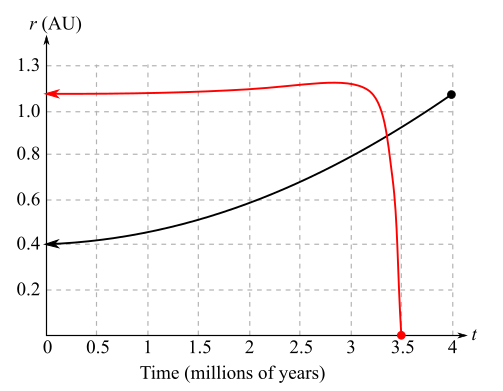Chapter 10.4, Problem 46EFinite Mathematics and Applied Cal...

7th Edition
Stefan Waner + 1 other
ISBN: 9781337274203

Solutions

Chapter
SectionFinite Mathematics and Applied Cal...

7th Edition
Stefan Waner + 1 other
ISBN: 9781337274203
Textbook Problem

End of the Earth In 5 billion year the Sun will have run out of hydrogen fuel and begin to expand into a red giant, eventually engulfing the Earth and causing it to spiral into the core of the Sun 7.5 billion years from now. The following graph50 shows the expanding radius of the red giant Sun (in red) and the radius of the Earth’s orbit about the Sun (in green) during its final three and a half million years of existence. The radii are measured in astronomical units (AU; one AU is the current radius of the Earth’s orbit around the Sun, approximately 93 million miles), and time is measured in millions of years.r (AU)The curve representing the Sun’s radius has equation r = 0.037 t 2 + 0.02 t + 0.4. ( t = 4 marks the end of the red giant expansion phase.) Exercises 45and 46are based on this curve.a. Calculate the rate of change of the radius of the Sun over the successive intervals [ 0 , 2 ] , [ 1 , 3 ] , [ 2 , 4 ] .b. The successive rates of change are a linear function of t. What is the slope of that linear function? How fast will the rate of change of the Sun’s radius be increasing in the final 4 million years?

(a)

To determine

To calculate: The rate of change of the radius of sun over the successive interval [0,2], [1,3], [2,4], where the graph shows the expanding radius of the red giant Sun whose radii have equation with respect to time is r=0.037t2+0.02t+0.4 where the radii are measured in astronomical units and time is measured in millions of years.Explanation

Given Information:

The graph shows the expanding radius of the red giant Sun whose radii have equation with respect to time is r=0.037t2+0.02t+0.4.

Where the radii are measured in astronomical units and time is measured in millions of years.

Formula used:

Average rate of change of f(x) over the interval [a,b] is:

Average rate of change of f=Change in fChange in x=ΔfΔx=f(b)f(a)ba

Calculation:

Considered the equation r=0.037t2+0.02t+0.4

Now, apply the average rate of change formula for the interval [0,2]:

Average rate of change of r=r(2)r(0)20

Compute the value of r(2) and r(0):

Now, substitute the value 2 in the function:

r(2)=0.037(2)2+0.02(2)+0.4=0.037(4)+0.04+0.4=0.148+0.04+0.4=0.588

Now, substitute the value 0 in the function:

r(0)=0.037(0)2+0.02(0)+0.4=0.037(0)+0+0.4=0+0+0.4=0.4

Substitute the value of r(2)=0.588 and r(0)=0.4:

r(2)r(0)20=0.5880.42=0.1882=0.094

Now, apply the average rate of change formula for the interval [1,3]:

Average rate of change of r=r(3)r(1)31

Compute the value of r(3) and r(1):

Substitute the value 1 in the function:

r(1)=0.037(1)2+0

(b)

To determine

To calculate: The slope of linear function r=0.037t2+0.02t+0.4 and interpret the answer if the graph shows the expanding radius of the red giant Sun where the radii are measured in astronomical units and time is measured in million of years.Still sussing out bartleby?

Check out a sample textbook solution.

See a sample solution

The Solution to Your Study Problems

Bartleby provides explanations to thousands of textbook problems written by our experts, many with advanced degrees!

Get Started

In problems 23-58, perform the indicated operations and simplify. 42.

Mathematical Applications for the Management, Life, and Social Sciences

Differentiate. y=ep(p+pp)

Single Variable Calculus: Early Transcendentals

The area of the shaded region is given by:

Study Guide for Stewart's Multivariable Calculus, 8th